# Search Results (26)

View
Selected filters:
• Fraction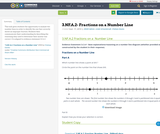Conditions of Use:
Remix and Share
Rating

This task gives students the opportunity to analyze two number lines in order to identify the one that correctly shows an improper fraction. Students then communicate their understanding by describing the reasoning they used to determine their answer was correct. It is aligned to evidence statement 3.C.6-1

Subject:
Education
Mathematics
Material Type:
Activity/Lab
Assessment
Homework/Assignment
Author:
Linda Schoenbrodt
Chelsea Davies
09/17/2018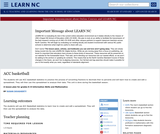Conditions of Use:
Remix and Share
Rating

The students will use ACC basketball statistics to practice the process of converting fractions to decimals then to percents and will learn how to create and edit a spreadsheet. They will then use this spreadsheet to analyze their data. This unit is done during the basketball season which takes approximately 15 weeks from the middle of November to the middle of March. Teachers must have Clarisworks to open the sample spreadsheet in the lesson, but may recreate it in another spreadsheet program.

Subject:
Statistics and Probability
Material Type:
Lesson Plan
Provider:
University of North Carolina at Chapel Hill School of Education
Provider Set:
LEARN NC Lesson Plans
Author:
Susan Dougherty
07/14/2000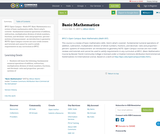Rating

BPCC Open Campus - Math 097: Basic Mathematics is a review of basic mathematics skills. Here's what's covered: -fundamental numeral operations of addition, subtraction, multiplication division of whole numbers, fractions, and decimals -ratio and proportion -percent -systems of measurement -an introduction to geometry NOTE: Open Campus courses are non-credit reviews and tutorials and cannot be used to satisfy requirements in any curriculum at BPCC.

Subject:
Education
Mathematics
Material Type:
Assessment
Full Course
Lecture
Lesson
Unit of Study
Author:
Allison Martin
10/11/2017Conditions of Use:
Rating

Subject:
Mathematics
Material Type:
Lecture
Provider:
PBS LearningMedia
Provider Set:
PBS Learning Media: Multimedia Resources for the Classroom and Professional Development
Teachers' Domain
Author:
U.S. Department of Education
WNET
07/08/2008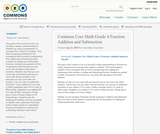Rating

One goal of the Common Core is to develop a deeper understanding of fractions by using a progression of concepts from simple to complex. This lesson prepares students for the conceptual shift involved in progressing from adding and subtracting whole numbers to adding and subtracting fractions. Students are guided to think of operations with fractions as very much like operations with whole numbers.
Students see that you can count with unit fractions just as you count with whole numbers. And because you can count with unit fractions, you can also do arithmetic with them. If you walked 2/5 of a mile (2 fifths) yesterday and 4/5 of a mile (4 fifths) today, altogether you walked 6/5 of a mile (6 fifths; because 2 things plus 4 more of those things is 6 of those things).
Students use the meaning of fractions and the meanings of addition and subtraction that were built in earlier grades to understand why the procedures for adding and subtracting fractions make sense.

Subject:
Mathematics
Material Type:
Lesson Plan
Author:
Curriculum Associates
01/28/2016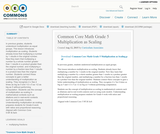Rating

In previous grades, students understood multiplication as equal groups.
This lesson introduces multiplication as scaling. Students already know that multiplying a number by 1 results in the original number. Now they learn that multiplying a number by a whole number greater than 1 results in a product greater than the original number, and multiplying a number by a fraction less than 1 results in a product less than the original number. Students connect these concepts to gain a better understanding of multiplication as scaling. They recognize 4 x 5as 4 times as big as 5 and 1/2 x 5 as 1/2 as big as 5 without performing computation.
Students use the concept of multiplication as scaling in mathematical contexts such as dilations and in real-world contexts such as using scale models. Understanding multiplication as scaling prepares students for Grade 6 work with ratios and proportional reasoning.
Aligned with Common Core 5.NF.B.5a/b

Subject:
Mathematics
Material Type:
Lesson Plan
Author:
Curriculum Associates
01/28/2016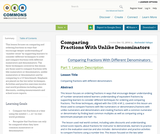Conditions of Use:
No Strings Attached
Rating

This lesson focuses on comparing and ordering fractions in ways that encourage deeper understanding of’ ‘number sense’ by supporting learners to consider different techniques to order and compare fractions with different numerators and denominators. The three techniques covered in this lesson are those used to compare fractions with like numerators or denominators, unlike numerators or denominators and by comparing to a 1/2 benchmark. Emphasis are placed on the two latter techniques. Activities and practice exercises involve real-world problems including sales discounts, cooking measurements and school score reports.

Subject:
Mathematics
Material Type:
Homework/Assignment
Lesson Plan
Author:
Rasheedat Yahaya
12/13/2016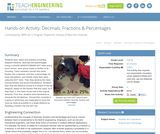Conditions of Use:
Rating

Students learn about and practice converting between fractions, decimals and percentages. Using a LEGO® MINDSTORMS® NXT robot and a touch sensor, each group inputs a fraction of its choosing. Team members convert this same fraction into a decimal, and then a percentage via hand calculations, and double check their work using the NXT robot. Then they observe the robot moving forward and record that distance. Students learn that the distance moved is a fraction of the full distance, based on the fraction that they input, so if they input ½, the robot moves half of the original distance. From this, students work backwards to compute the full distance. Groups then compete in a game in which they are challenged to move the robot as close as possible to a target distance by inputting a fraction into the NXT bot.

Subject:
Engineering
Mathematics
Material Type:
Activity/Lab
Provider:
TeachEngineering
Provider Set:
TeachEngineering
Author:
Javed Narain
09/18/2014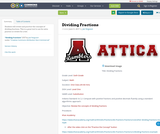Conditions of Use:
Remix and Share
Rating

Students will review and practice the concepts of dividing fractions. This is a great tool to use for extra practice or review for a test.

Subject:
Mathematics
Material Type:
Activity/Lab
Author:
Joe Ferguson
06/09/2017
Remix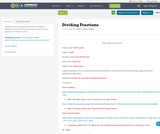Conditions of Use:
Remix and Share
Rating

Students will review and practice the concepts of dividing fractions. This is a great tool to use for extra practice or review for a test.

Subject:
Mathematics
Material Type:
Activity/Lab
Author:
Dylan Shaffer
10/16/2019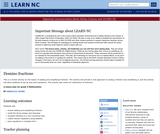Conditions of Use:
Remix and Share
Rating

This is a review activity on the lesson of adding and simplifying fractions. This activity will provide a new approach to seeing a fraction and simplifying it, and the activity will allow students to set up and solve equations. This activity also works for subtraction of fractions.

Subject:
Mathematics
Material Type:
Lesson Plan
Provider:
University of North Carolina at Chapel Hill School of Education
Provider Set:
LEARN NC Lesson Plans
Author:
Angelica Young
10/13/2000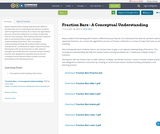Conditions of Use:
Remix and Share
Rating

Many students find working with fractions difficult because they do not understand the abstract symbols used to represent fractions. As a result, the algorithmic process of fraction arithmetic is a series of steps that have no real meaning.

This training will teach students how to use fraction bars to gain a conceptual understanding of fractions. This conceptual understanding will help the student build a strong foundation for a traditional in-depth study of fractions.

Participants will use fraction bars to add, subtract, multiply, and divide fractions. Lessons include student practice sets designed to maximize instruction by creating an environment where students actively participate in the learning process.

Subject:
Mathematics
Material Type:
Lesson
Author:
Larry Stacy
01/18/2017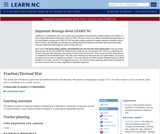Conditions of Use:
Remix and Share
Rating

This lesson plan introduces a game that will reinforce fractions and decimals. This activity is best played in groups of 3-4. You may choose to use as an activity when work is completed or as a center activity.

Subject:
Mathematics
Material Type:
Lesson Plan
Provider:
University of North Carolina at Chapel Hill School of Education
Provider Set:
LEARN NC Lesson Plans
Author:
Christine Sisco
06/25/1999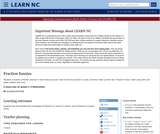Conditions of Use:
Remix and Share
Rating

Students complete a fraction exercise in which they are given clues to find the answer to a joke, and then make up their own "Fraction Funny" sheet using their own jokes (riddles) and clues. Students will demonstrate their knowledge of fractions as "parts of a whole" and of fractions in lowest terms.

A lesson plan for grade 4 Mathematics

Subject:
Mathematics
Material Type:
Lesson Plan
Provider:
University of North Carolina at Chapel Hill School of Education
Provider Set:
LEARN NC Lesson Plans
Author:
Alisan Royster
06/25/1999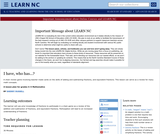Conditions of Use:
Remix and Share
Rating

A chain drill involving teacher made cards on the skills of adding and subtracting fractions, and equivalent fractions. In this chain activity the student chosen to begin the chain will read the card aloud and then wait for the next participant to read the only card that would correctly follow the progression. Play continues until all of the cards are read and the initial student is ready to read his card for the second time.

Subject:
Mathematics
Material Type:
Lesson Plan
Provider:
University of North Carolina at Chapel Hill School of Education
Provider Set:
LEARN NC Lesson Plans
Author:
Sherry Russell
01/30/2001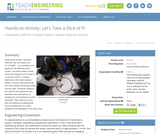Conditions of Use:
Rating

Working as a team, students discover that the value of pi (3.1415926...) is a constant and applies to all different sized circles. The team builds a basic robot and programs it to travel in a circular motion. A marker attached to the robot chassis draws a circle on the ground as the robot travels the programmed circular path. Students measure the circle's circumference and diameter and calculate pi by dividing the circumference by the diameter. They discover the pi and circumference relationship; the circumference of a circle divided by the diameter is the value of pi.

Subject:
Engineering
Mathematics
Material Type:
Activity/Lab
Provider:
TeachEngineering
Provider Set:
TeachEngineering
Author:
Carole Chen
Michael Hernandez
09/18/2014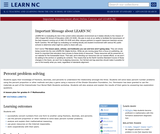Conditions of Use:
Remix and Share
Rating

Students apply their knowledge of fractions, decimals, and percents to understand the relationship amongst the three. Students will solve basic percent number problems using the percent proportion or other methods and play a game using a resource of the Shodor Education Foundation, Inc. Permission has been granted to use the materials as part of the Interactivate Your Bored Math Students workshop. Students will also analyze and explain the results of their game by answering two exploration questions.

Subject:
Mathematics
Material Type:
Lesson Plan
Provider:
University of North Carolina at Chapel Hill School of Education
Provider Set:
LEARN NC Lesson Plans
Author:
Victoria Lunetta